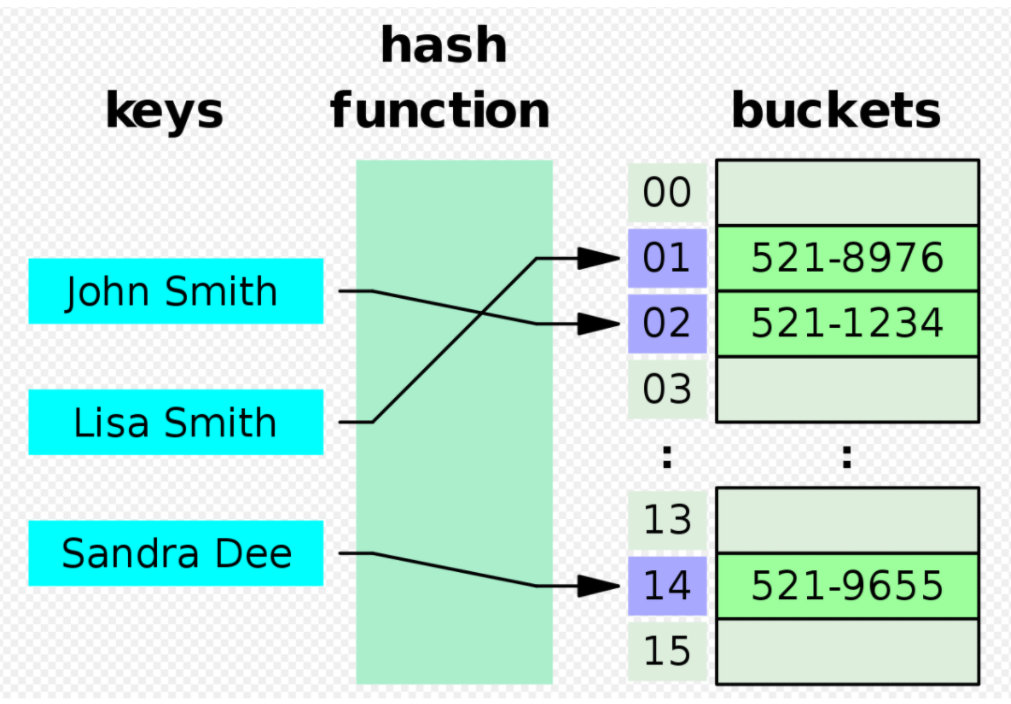# Hash Table

Hash tables are a type of data structure in which the address/ index value of the data element is generated from a hash function. This enables very fast data access as the index value behaves as a key for the data value.

In other words, hash tables store key-value pairs but the key is generated through a hashing function. So the search and insertion function of a data element becomes much faster as the key values themselves become the index of the array which stores the data. During lookup, the key is hashed and the resulting hash indicates where the corresponding value is stored.

## Hash Table Architectural OverviewThe idea of hashing is to distribute the entries (key/value pairs) across an array of buckets. Given a key, the algorithm computes an index that suggests where the entry can be found:

`index = f(key, array_size)`

Often this is done in two steps:

`hash = hashfunc(key)`

`index = hash % array_size`

In a well-dimensioned hash table, the average cost (number of instructions) for each lookup is independent of the number of elements stored in the table. Many hash table designs also allow arbitrary insertions and deletions of key-value pairs, at constant average cost per operation.

In many situations, hash tables turn out to be more efficient than search trees or any other table lookup structure. For this reason they are widely used in many kinds of computer software, particularly for associative arrays, database indexing, caches, and sets.

## Hash Tables in Data Science

Hash tables allow a data scientist to take an arbitrary value, such as a string, a complex object or a data frame, and use it as a lookup to find another value. Common uses for hash-table data structures in the data cleaning and preparation phase include feature engineering (e.g., keeping a count of how many times you’ve seen an individual value in a stream), normalization, or even creating simple histograms.

In Python, the Dictionary data types represent the implementation of hash tables. The Keys in the dictionary are hashable–they are generated by a hash function which generates a unique result for each unique value supplied to the hash function. Also, the order of data elements in a dictionary is not fixed.

In R, if you need to store key value pairs, and your keys are always going to be valid R symbols, the built-in `new.env(hash=TRUE)` is the best approach.